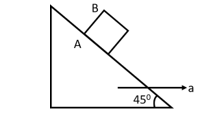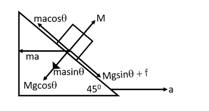Variation of friction with applied force
Question

# If the coefficient friction between A and B is $\mathrm{\mu }$ , the maximum acceleration of the wedge A for which B will remain at rest with respect to the wedge isDifficult
Solution

## For maximum acceleration body as a tendency to go up and friction acting down the plane.$\mathrm{macos\theta }=\mathrm{mgsin\theta }+\mathrm{f}$$\mathrm{macos\theta }=\mathrm{mgsin\theta }+\mathrm{\mu }\left(\mathrm{masin\theta }+\mathrm{mgcos\theta }\right)$$\mathrm{a}=\mathrm{g}\left(\frac{1+\mathrm{\mu }}{1-\mathrm{\mu }}\right)$

Get Instant Solutions